Published: 17 August 2022

# The application of seismic parameters conversion among different structure design codes

Qi Xie1
1GBC Engineers (Cambodia) Co., LTD, No. 24 Street 331, Sangkat Boeng Kak II, Khan Toul Kork, Phnom Penh, Kingdom of Cambodia
Views 63

#### Abstract

The main goal for structure engineer is to do safety design and make sure structures can resist seismic forces by adopting proper construction materials and structure systems. The seismic design parameters are the key factors to control the structure seismic design procedures and results. These parameters covered several items, such as seismic spectral response acceleration in 1 second (S1) and short period (SS), site classification(A~E), sites importance (Ie,), strong motion files with their pseudo-acceleration and displacement, etc. Around these parameters, different countries and regions also issued the domestic design principles correspondence. For international projects, no matter which area or country projects located in, every structure engineer should take more care about the proper seismic parameters to deploy seismic design, and the issues of seismic parameter conversion among various codes appeared a few decades ago. In this article, author focused on multiple stories hotel (RC) structure design with GB and ASCE seismic parameters controlling, demonstrate the difference of structure design principles between GB and ASCE, and applied specified seismic parameters conversion method, then deploy structure analysis based on these parameters, got series structure analysis results. In the end, author provided a few practical advice about developing strategies and decisions from consultant view. These conclusions not only can benefit developers, also strengthen the support from consultant agency, besides, the parameter screen procedures enhanced the structure designer’s driving ability on various structures which locate in different regions and countries.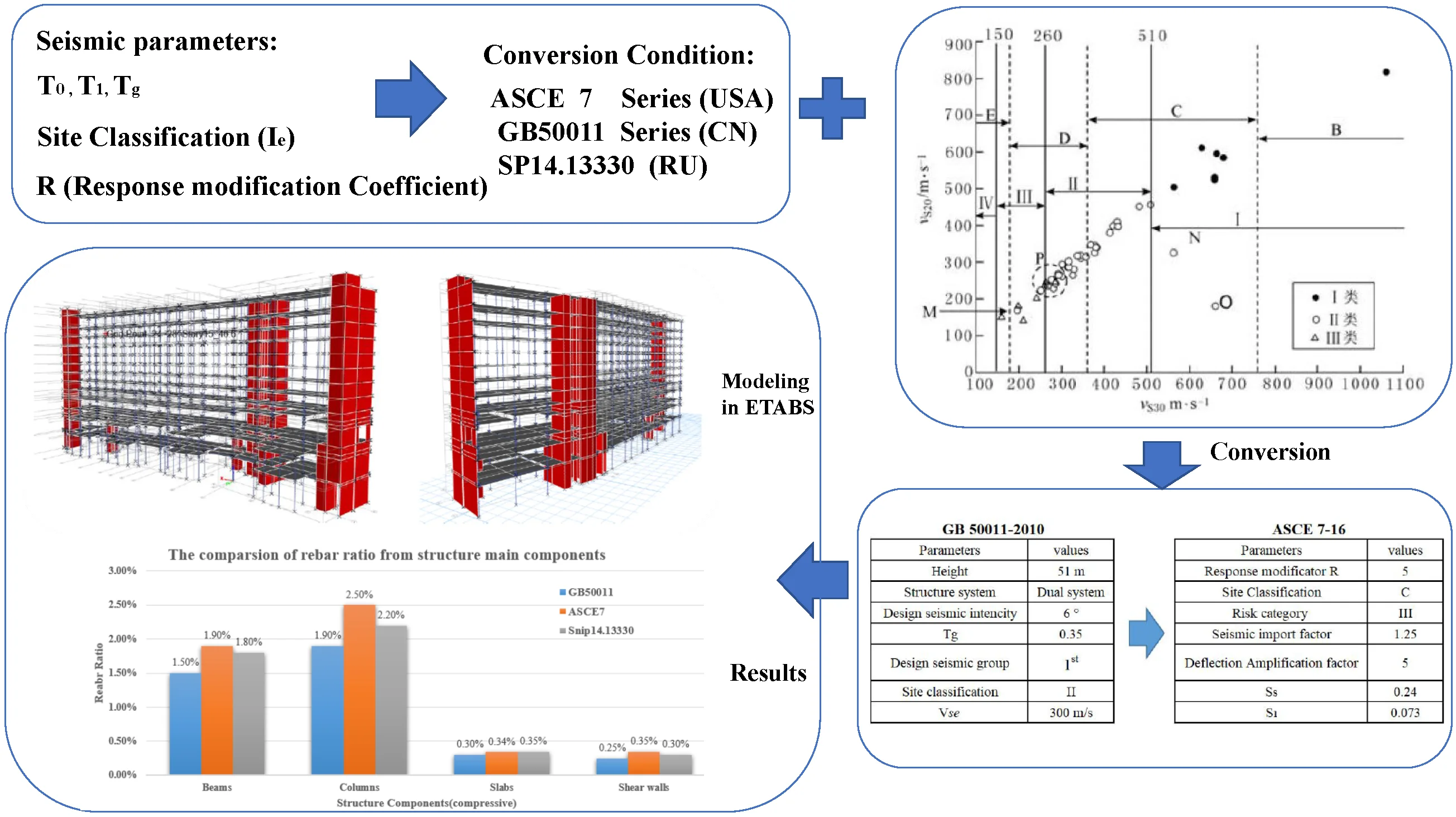#### Highlights

• Seismic parameters
• R (Response modification Coefficient)
• Site Classification (Ie)
• Conversion condition

## 1. Introduction

The conducting and confirmation about the proper seismic design parameters are the key factor to perform a full structure analysis for every structure engineer, also it is the core interests and critical concern for project’s investors, as the demands of construction development in various countries and districts, it is common that structure engineer will face the problem that, in order to estimate the investment budget based on the origin fund, and fit the domestic construction cost, not only adopt local design codes, also investors request for the structure engineer should take other recognized design codes and criteria to compare with the local design results. Therefore, the problems of evaluation criteria and parameters conversion stand in front of structure designer. Author here demonstrated a practical case (12F RC building) which highly demands of the design parameters conversions between different codes from investors, this project is invested by some Southeast-Asia investment group (regulated by US design codes), but located in Russia territory, where is very closed to Chinese border as well, the design and construction are carried out by Chinese main contractor. Due to the cost estimation, investors need two versions structure design schemes (based on GB and ASCE), permitted by local authorities, it is allowable to adopt Chinese GB codes to design the structures and verified by Russia design agency. Under this condition, structure engineer should convert seismic parameters from Snip14.1330  and GB50011  to ASCE7 codes before the structure design commence. Hereby, to achieve this goal, it is necessary to review the present research methodology of seismic parameter design among these codes.

## 2. Codes principal and demonstration

The most popular and wildly applied seismic codes are ASCE7, ASCE41, IBC (International Building Codes)  series, which are developed from BSSC (Building seismic safety committee), NEHRP (National Earthquake Hazards Reduction Program). The project location, Russia also regulated series seismic design codes, such as SP14.13330, consequent Chinese authorities combined partial principles from both above, and developed GB50011, GB18306  for Chinese seismic region projects. Without any exception for seismic design, all structure engineer frequently touched parameters, which are ${S}_{S}$, ${S}_{1}$(spectral response acceleration parameter), and site classification, site importance Coefficient ${I}_{e}$, and response modification coefficient $R$.

### 2.1. The comparisons of structure design procedure:

In ASCE7-16: To determine the seismic risk category of buildings, such as A, B, C, D, E, F, 6 types, structure engineer will confirm them from classification of building function (I, II, III, IV) and design ground motion parameters (${S}_{DS}$ or ${S}_{D1}$), Class ‘A’ structures require the basic seismic measures; Class B and C require general seismic measures, generally only need to analyze the seismic loads based on the static method-Equivalent Lateral Force (ELF), and the demand of structural ductility ($R$) requirements lower than other type; Classes D, E and F are required to satisfy the requirements of seismic measures strictly, in the meantime, it is mandatory to complete the time history analysis (Modal Response Spectral Analysis, MRSA, etc.) and the base shear forces from MRSA are not less than 85 % of the ELF results, also mean that the structural ductility ($R$) requirements are higher than others relatively, $R$ is critical index for dissipating energy as well, which are illustrated by ‘hysteresis curves’ generally, such as Elastic type, Kinematic type , Takeda type , BRB hardening type , etc.

In GB and Snip codes systems, they have the similar design concept, therefore author cites GB codes to express Snip series codes main concepts, in the practical phase, their analyzed results are very close to each other. besides, permitted by local authorities and demands from investor, all parties agree to adopt GB codes to design and verified by Snip codes. In terms of seismic design, especially after the Wenchuan earthquake (M.8.0) in 2008, GB50011 series codes revised and take the some similar procedures from the ASCE7, the obvious discrepancy  between them is the GB50011 codes adopted much more seismic measures to strengthen the seismic resistance capacity of structures without considering the importance factor (Refer. Comments 5.4.1.4), but ASCE7 does and controlled by $R$ value mostly.

### 2.2.1. Site classification definitions

In ASCE7, it is pointed out that the average shear wave velocity (${V}_{s30}$) within 30 m of the surface layer is mainly used to divide site classification into 6 categories (A, B, C, D, E, F). the internal conversion based on the ‘B’ class.

However, in GB50011, the specification points out that the site category is divided into 4 categories (I (${I}_{0}$, ${I}_{1}$), II, III, IV) according to the equivalent shear wave velocity (${V}_{s20}$) of the rock and soil layer within the depth range of 20 m below the surface and the thickness of the site cover, the internal conversions based on ‘II’ class.

In Snip codes, it points out that site category can be divided into (I, II, III) with the equivalent shear wave velocity (${V}_{s10}$) of the rock and soil layer within the depth range of 10 m below the surface and the thickness of the site cover. under the permission from investor and local authorities, it is allowable for Chinese design teams to choose the GB seismic parameters, the results from GB and Snip fit well in this region, therefore, author only do the GB to ASCE conversion.

The conversions of the site category are carried out according to the equivalent shear wave velocity, as per Lu’s transformation method , the dependency figure showing in Fig. 1.

Fig. 1Comparison of site category in GB50011 and ASCE7 code (From Lu  Fig. 1)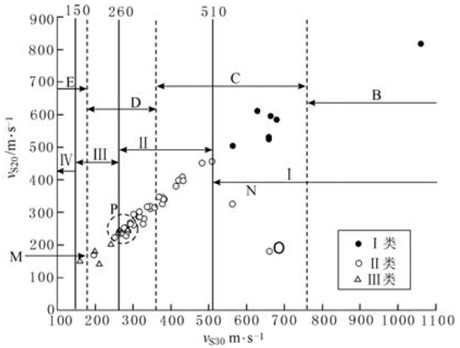### 2.2.2. Design site seismic period conversion and the damping ratio

For site characteristic period, it is known that final purpose of the classification of the site is to determine the design characteristic period of the site (marked as ${T}_{g}$, in GB codes). not only the characteristic period of the seismic influence coefficient related to the site category, also to the design seismic group, which can better reflect the influence of seismic magnitude, epicentral distance and site conditions. According to the GB18306-2015, the ${T}_{g}$ values listed below:

Table 1Characteristic period of site Tg (GB50011-2010, Tab.5.1.4-2)

 Design seismic group Site Classification I0 I1 II III IV 1st Group 0.2 0.25 0.35 0.45 0.65 2nd Group 0.25 0.3 0.4 0.55 0.7 3rd Group 0.3 0.35 0.45 0.65 0.9

In ASCE7-16, ${T}_{s}={S}_{Ds}/{S}_{D1}$, ${T}_{0}=0.2\left({S}_{Ds}/{S}_{D1}\right)$, they define the. For long period, ${T}_{L}$ can be conducted from as per ASCE7-16 (11.4-7), in this case, ${T}_{L}=$ 4.0 s.

In the practical phase, the damping ratio in Snip14.13330, GB50011 and ASCE7 codes are the same equal to 5 %, therefore, no need to conversion.

### 2.2.3. From the aspect of seismic time dimension

In GB codes, it is accepted that engineer use the basic seismic intensity to evaluate the basic seismic (50-year probability of exceedance is 10 %), however in ASCE7, the maximum seismic force (50-year probability of exceedance is 2 %) represent the basic seismic force, we use ${\gamma }_{CN}$ coefficient to bridge two codes.

The response spectrum acceleration in GB50011 is the ground peak acceleration, however, the response spectrum acceleration in ASCE7 represents the response acceleration on the structure, so we import structural influence coefficient ${C}_{s}$.

The Eq. (1) that GB coefficient convert to ASCE coefficient concluded below:

1
${S}_{s}=\frac{{C}_{s}{\gamma }_{CN}{A}_{cc}}{{F}_{a}},$
${S}_{1}=\frac{{C}_{s}{\gamma }_{CN}{A}_{cc}{T}_{g}}{{F}_{v}},$

where, ${C}_{s}$ is structural coefficient 2.5 from Yu ; ${S}_{s}$ and ${S}_{1}$ are the spectral response acceleration parameter at short periods and 1s in ASCE7; ${F}_{a}$ and ${F}_{v}$ are the site coefficients based on ASCE7, we value them as “1” (constant) as out of USA; ${A}_{cc}$ is the peak acceleration of seismic ground motion corresponding to basic seismic intensity; ${T}_{g}$ is the site characteristic periods based on GB50011; ${\gamma }_{CN}$ is the ratio of peak acceleration for each return period (2474y / 475y).

Table 2Ratio of peak acceleration for each return period γCN

 50-year probability of exceedance Return 6 Degree 7 Degree 7 Degree 8 Degree 8 Degree 9 Degree (Y) ${A}_{cc}$ (0.05 g) ${A}_{cc}$ (0.1 g) ${A}_{cc}$ (0.15 g) ${A}_{cc}$ (0.2 g) ${A}_{cc}$ (0.25 g) ${A}_{cc}$ (0.4 g) 63.20 % 50 0.35 0.35 0.37 0.35 0.37 0.35 10 % 475 1 1 1 1 1 1 2.50 % 1975 - 2.51 2.31 2 1.7 1.5 2 % 2474 2.5 2.97 2.69 2.25 1.84 1.58

## 3. The conversion procedure from GB50011 to ASCE7

Seismic Basic Parameters in GB format and transferred ASCE results listed in Fig. 2, the conversion procedures recorded in 3.1 and 3.2.

Fig. 2GB-ASCE conversion results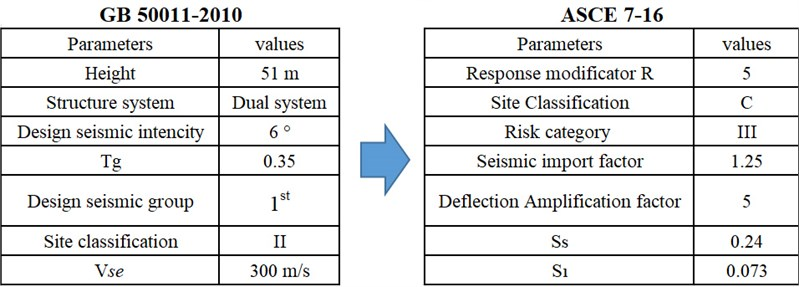### 3.1. Site classification conversion

From the geotechnical report, refer GB50011-2010 (equation 4.1.5.1/2), we can conduct the equivalent shear velocity at depth = 20 m, ${V}_{S20}=$20/(10/300+10/500) = 375 m/s > 330 m/s, refer Fig. 1, we can conclude this site belongs to ‘C’ class according to ASCE7 code.

### 3.2. ${\mathbit{S}}_{\mathbit{s}}$ and ${\mathbit{S}}_{1}$ parameter conversion

From Table 2, we can find the ${\gamma }_{CN}$= 2.5, ${A}_{cc}$= 0.05, ${F}_{a}$ = ${F}_{v}$= 1, and substitute them into Eq. (1), and do loop iterations on ${S}_{s}$ and ${S}_{1}$, to make sure the ${\left(S}_{s}-{S}_{s}\text{'}\right)/{S}_{s}$ and ${\left(S}_{1}-{S}_{1}\text{'}\right)/{S}_{1}$ two results all less than 0.5 % in the same iteration step, then lock the latest ${S}_{s}=$ 0.24, and ${S}_{1}$= 0.073.

## 4. 4The application on the practical model and results analysis

This project design commenced at 2016 year, with three blocks: they are 12F reinforcement concreate (RC) structure ($H=$ 52.2 m), 3F RC (Entertainment zone), 3F RC + Steel truss (Ballroom), in this case, we only discuss the high-rise RC building (12F), the numerical models established in Fig. 3.

Fig. 312F Guest Hotel Block Layout and Elevation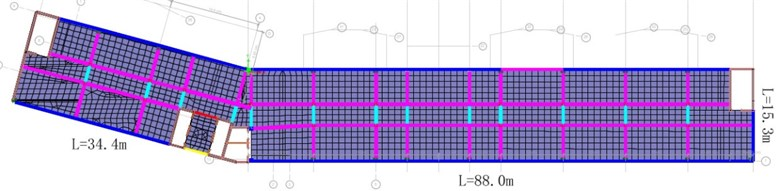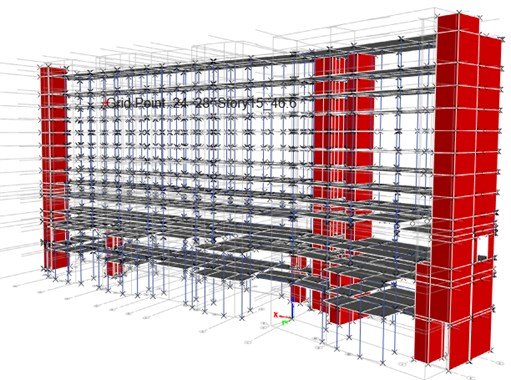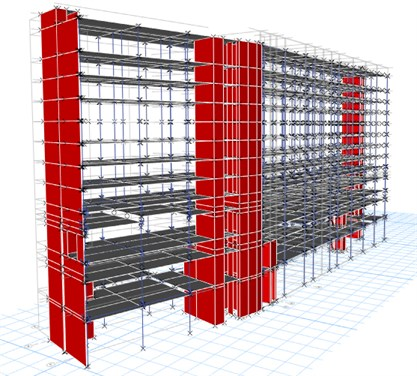As per ASCE7 and GB50011, for the dual system, it is necessary to perform the time history analysis, ASCE7-16 requires the number of ground motion records should more than 7 lists at least in ASCE7-16 (С16.2.2), the mean values of them can evaluate the seismic analysis, but in previous edition ASCE7-10, only 3 records for non-linear response analysis. Li and Wen  also proposed the similar conclusions that more than 7 records can promote the guaranteed probability of strong motion ground to 84 %. In this case, for developer strategies, we adopt the exist and well recognized strong motion records with 5 % damping value from Berkeley (PEER Ground Motion Database) and match this site seismic parameters  by SeismoMatch . The matched depicts showing in Fig. 4.

Fig. 4Matched accelerogram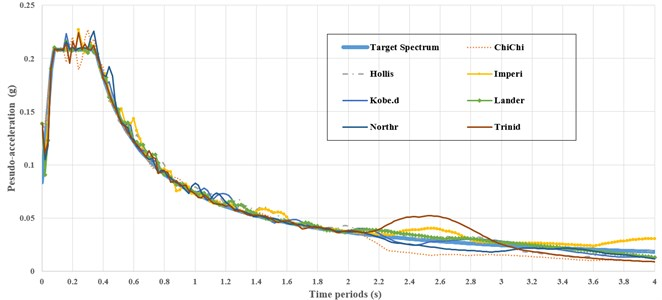After the time history analysis on this dual system structure, it is easy to find the static charts of rebar ration, which calculated based on these three codes, the results are shown in Fig. 5.

Fig. 5The comparison of rebar ratio from structure main components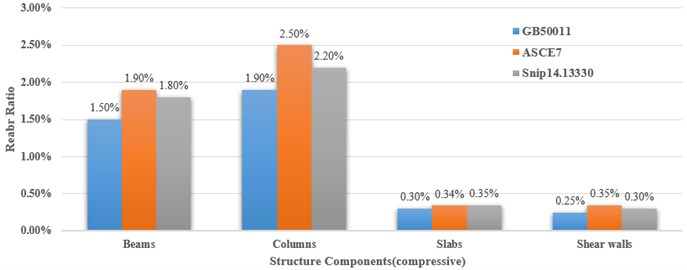## 5. Conclusions

Based on the understanding of the GB50011, Snip14.13330 and ASCE7, from this case, author here proposes some practical advice for potential project developer, meanwhile, main contractors also can be benefit from them, especially for the investment dynamic management.

1) This article provides an approach of seismic parameters conversion from GB codes to ASCE codes, structure engineer can refer these parameters and control the procedure and results for clients’ specified demands, different relevant parties will take their own strategies.

2) Based on the construction phase and final “as-build” verification, the structure analysis and results from GB codes are similar with Snip codes performance, as most of GB codes system developed from Snip system since the GB birth.

3) In terms of amount of rebar consumption on this high-rise building (upper structure), when structure engineer takes the equivalent seismic parameters which are converted from GB to ASCE codes, ASCE codes results showed mostly larger than Snip and GB codes calculated, it is mainly caused by the different structure design concepts.

4) The chosen of seismic parameters must authorized by project local official administrative agency, although this method have been verified by consultant agency and main contractor, hence, it is suggested that the conversion method can be applied on internal enterprise decision.